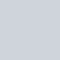# 先來看看今天的結果圖## -> 此篇文章的程式碼 github

Day11_建立新空白圖畫點畫圓_new_pictures_point_circle.ipynb

# 運用 OpenCV 建立新空白圖、畫點、畫圓

## 建立新空白圖

`因為全部的值都是0，所以圖片是全黑的`

``````# 建立全黑的新圖片 100*100
shape = (100, 100, 3) # y, x, RGB
origin_img = np.zeros(shape, np.uint8)
``````### 那要怎麼建立全白的圖片呢？

``````shape = (100, 100, 3) # y, x, RGB

# 第一種方法，直接建立全白圖片 100*100
origin_img = np.full(shape, 255).astype(np.uint8)

# 第二種方法，一樣先建立全黑的圖片，再將全部用白色填滿。
origin_img = np.zeros(shape, np.uint8)
origin_img.fill(255)
``````## 運用 OpenCV 畫點

``````def draw_points(img):

point_size = 1
point_color = (0, 0, 255) # red
thickness = 4

# 要畫的點座標
points_list = [(80, 20), (20, 80)]

for point in points_list:
cv2.circle(img, point, point_size, point_color, thickness)

return img
``````

cv2.circle(img, point, point_size, point_color, thickness)
* img 原圖片
* point 要畫的點座標
* point_size 點的大小
* point_color 點的顏色
* thickness 點的粗細(這裡值類似點的大小的效果)## 運用 OpenCV 畫圓

``````def draw_cirlces(img):
circle_mid = (80, 80)
color = (0, 255, 0) # green
cv2.circle(img, circle_mid, 10, color, 0)

# 黃色圓圈，線條寬度為 3 px
cv2.circle(img,(30, 30), 30, (0, 255, 255), 3) # yellow

# 藍色實心圓圈
cv2.circle(img,(50, 50), 15, (255, 0, 0), -1) # blue

return img

``````

cv2.circle(img, point, point_size, point_color, thickness)
* img 原圖片
* point 要畫的圓圓心
* point_size 圓的大小 (也就是`半徑`)
* point_color 圓的顏色
* thickness 圓的線條粗細， `-1 表示將圓填滿顏色`【沒錢ps,我用OpenCV!】Day 11 – 基本修圖3，OpenCV 建立新空白圖、畫點、畫圓 create new pictures, draw points and draw circle

# Reference

https://docs.scipy.org/doc/numpy-1.13.0/reference/generated/numpy.full.html

Python 與 OpenCV 基本讀取、顯示與儲存圖片教學

https://blog.csdn.net/u011520181/article/details/83933325

Python 與 OpenCV 加入線條圖案與文字教學

https://blog.gtwang.org/tag/opencv/

https://www.geeksforgeeks.org/python-opencv-cv2-circle-method/### 1 則留言

1. #### 【PyQt5】Day 17 / Project 製作標註 roi 工具, 開始導入 OpenCV 作為繪圖引擎, 在圖上畫點並顯示座標 - 嗡嗡的隨手筆記

[…] 不知道怎麼使用 OpenCV 畫點? 可以參考我的另外一篇文，內有詳細說明：【OpenCV】11 – OpenCV 建立新空白圖、畫點、畫圓 create new pictures, draw points and … […]